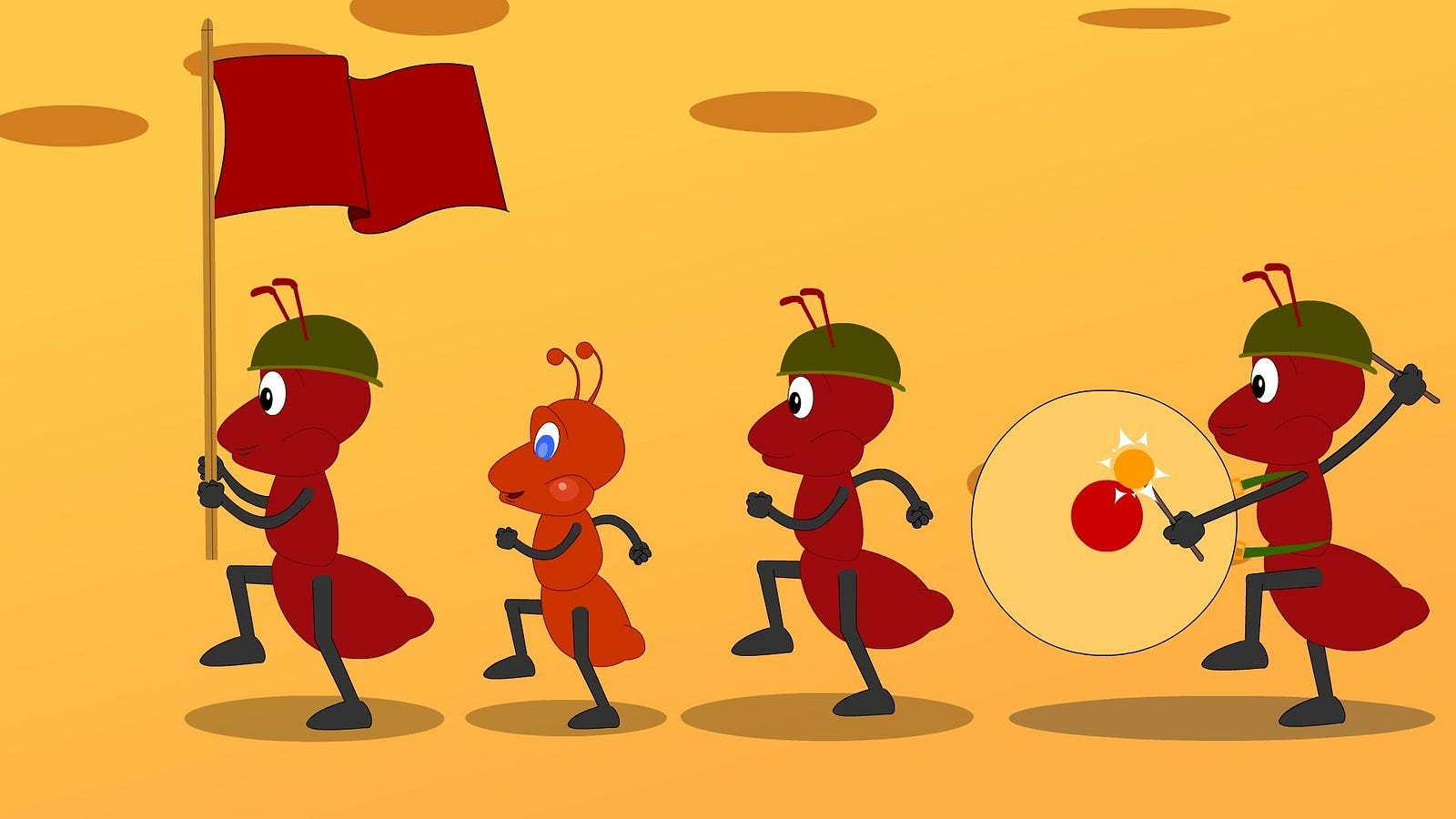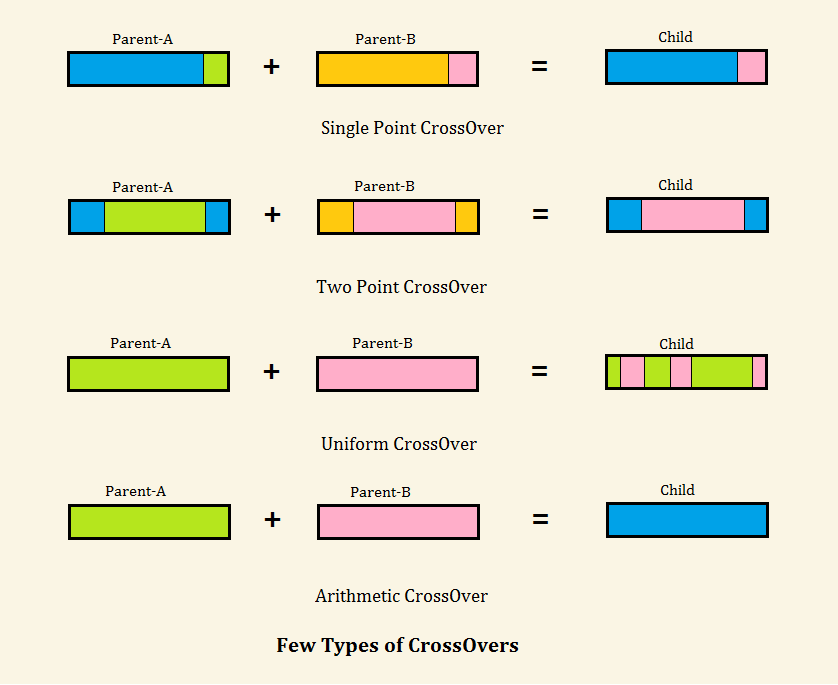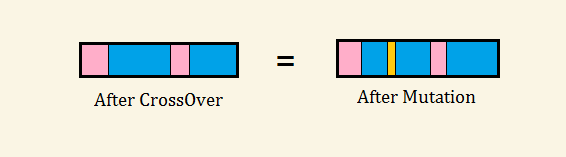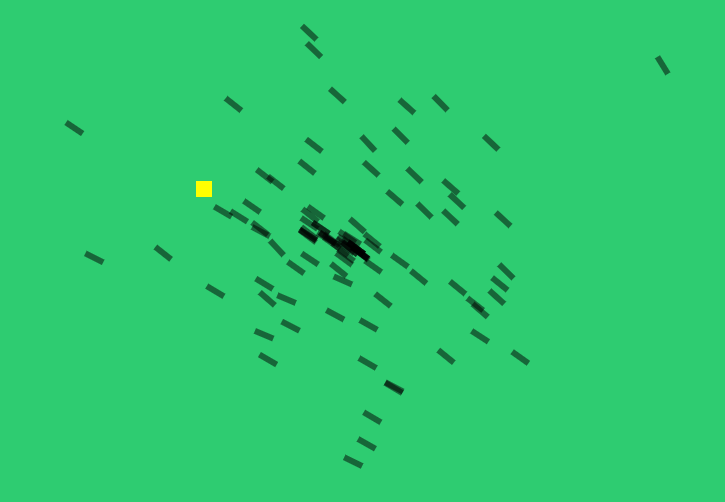# Understanding Genetic Algorithm

If we are solving some problem, we are usually looking for some solution, which will be the best among others. The space of all such feasible solutions is called ‘search space’, each point in the search space represent one feasible solution. We are looking for our solution, which is one point (or more) among all feasible solutions.

The problem is that the search can be very complicated. One does not know where to look for the solution and how to start. This is where Genetic Algorithm comes into play, let us know about it with one simple example.

Basic Genetic Algorithm: (just have a look at it)

`step1: (Initialize)`
`     Create a population of N elements, each with randomly generated DNA.`
`step2: (Selection)`
`     Evaluate fitness of each element of the population and build a mating pool.`
`step3: (Reproduction)`
`     Repeat this step N times.`
`         a. Pick two parents with higher probability, according to relative fitness.         b. Crossover — create a child by combining the DNA of two parents.         c. Mutation — mutate the child’s DNA based on given probability.         d. Add the new child to new population.`
`step4:`
`Replace the old population with new population and return to step2.`

Don’t worry much about understanding this Algorithm, let us apply it to create one simple Application so that we can understand it better.

Theme of the Application:

There are finite number of ants starting at some place, their goal is to reach a sweet (target) located at some location. This can be done using Genetic Algorithm with several iterations,

i.e., each time an ant knows some information about the location of sweet from some random ant, it will share that information with its children and dies. In this way every time they get to know more information, they will start moving towards the location. Let us implement the code for this using html5 and JavaScript, canvas will act like space in which both sweet and ants are present.Ants walking happily towards the Sweet (Target)

First of all, let us create an Ant:

Oops! I am not that good at designing, so for now, let us assume a rectangle as an ant.

Drawing rectangle:

`draw_ant(x,y,a){    translate(x,y);    rotate(a);    fill(255,0.5);    rect(0,0,25,5);}`

draw_ant will take three arguments in which x,y represents position of an ant in canvas, ‘a’ represents angle at which ant is looking.

Translate() — will translate canvas cursor position to (x,y).

Rotate() — will rotate canvas cursor in a given angle, which is the direction in which the ant is looking.

Fill() — will set color to draw with opacity 0.5 , opacity is taken as 0.5 to observe if one ant is moving over another ant.

Rect() — this will ideally draw rectangle with height 25 and width 5 at (0,0) but it draws at (x, y) with ‘a’ rotation in canvas as we have already translated to (x, y) and rotated with an angle.

Wow! we can now use this function to draw an ant whenever and where ever we like to.

Adding properties to ant object:

An Ant is a movable physical object it should have a position, velocity, acceleration.

In order for an ant to move by itself and to communicate with other ants it should have brain which means it should be having some DNA. Is that right! Isn’t scary to imagine the creation of DNA for a living creature, so let’s start with what we know. We know that DNA is set of ‘genes’.

So let us assume that every ant has single DNA with some constant number of genes, here each ‘gene’ specifies an ant’s movement for each step it takes.

So, before creating an ant we must create DNA object that contains n genes.

`function DNA(){    var genes = [];    for(var i=0;i<lifespan;i++){        genes.push(randomeVector())    }}`

Lifespan -> lifespan is number of steps an ant can take in its whole life time. (i.e., distance it can travel in its whole life).

We are using vector here, as the field in which the ant is moving is two dimensional.

`function Ant(){    var pos;    var vel;    var acc;    var dna;    var fitness;`
`    getFitness();    showAnt();    update();    applyforce();}`

pos, vel, acc are vectors and define their corresponding property in two dimensional space.

‘fitness’, what is this new variable?

Yes, it is the most important variable for every application which uses genetic algorithm. In our application ant’s fitness will be in the range of 0–1, the higher this value, the nearer the ant is to the sweet.

so how to calculate the fitness?

It is very simple , just find the distance between sweet and the ant.

`getFitness(){    var d = distance_between_points(this.pos,target);    return 1/d;}`

‘update’ -> this function updates the position of ant, whenever we want it to.

`update(){    this.applyforce(this.dna.genes[k]);    this.vel.add(this.acc);    this.pos.add(this.vel);    this.acc.mul(0);}`

‘applyforce’ -> this function applies genes[‘k’] force to the ant, so that ant will take a k’th move.

`applyforce(force){    this.acc.add(force);}`

So using above ant class, we can create ant, we can move ant, we can show ant in any location, looking at any direction.

Hurray! now we are done with most of the code.

Let us think about how the ants communicate and how they transfer the known information to their child. ‘Crossover’, ‘mutation’ will now come into action.

what is Crossover?

In Crossover two ants talk to each other and will give some of their properties to a (new/another) ant.

suppose there are two ants say ‘ant-A’,’ant-B’

genes in ant-A -> [1, 2, 3, 4]

genes in ant-B -> [a, b, c, d]

then, in our case new ant will get a hybrid gene = [1, 2, c, d] or [1, b, c, d] or [1, 2, 3, d]…

Code for crossover:

`crossover(partner_dna){    var crossover = Math.floor(getRandom(this.genes.length));    var hybrid_genes=[];    for (var i = 0; i < this.genes.length; i++) {        if (i > crossover)            hybrid_genes[i] = this.genes[i];        else            hybrid_genes[i] = partner_dna.genes[i];    }    var newdna = new DNA(hybrid_genes);    return newdna;}`CrossOver Types

What is Mutation?

Before knowing about mutation, let us know about drawback in crossover.

Using only crossover, next generation will get to know only what the previous generation knows, but what if this happens in real life?

OMG! we might not be able to read this very blog , as our previous generation didn’t even think of having a Script for a language.

Mutation is a simple concept in which new characteristics which are independent of its previous generation will be added to the next generation. In our application new characteristics are ‘random vectors’ assigned to very few genes in few ants.

Code for mutation:

`mutation(mutationRate){    for (var i = 0; i < this.genes.length; i++) {        if (getRandom(1) < mutationRate) {            this.genes[i] = createRandomVector();        }    }}`

‘mutationRate’:

• It defines the probability of adding new characteristics, more the value, more the number of new characteristics added to the next generation.
• The range is ‘0 to 1’

NOTE: It is not preferrable to have either higher mutationRate or 0.Mutation
Till now, we have a complete functionality of an ant.

Step1: (Initialize)

As we are able to create a complete Ant, now we have to create army of ants so, here is the code to create population:

`for(var i=0;i<this.popsize;i++){    this.ants[i]=new Ant();}`

‘popsize’ is number of ants in each generation, this is our choice.

Step2: (Selection)

This is the step where we select ants for generating the next generation of ants. Selection will be based on ant’s fitness, which we have already discussed before. More the fitness, more the chances the ant has to pass on the information to next generation.

`selection(){    var maxFitness=this.getMaxFitness();    this.matingPool = [];    for (var i = 0; i < this.ants.length; i++) {        var fitness = this.ants[i].fitness/maxFitness;        var n = Math.floor(fitness * 100);  // Arbitrary multiplier        for (var j = 0; j < n; j++) {            this.matingPool.push(this.ants[i]);        }    }}`

We multiply fitness with 100 but for our convenience let us assume we multiply by 10 as the fitness value ranges from 0 to 1,

Say ant-A is with fitness 0.9 after multiplying with 10, it will be 9, that means it will be added to the mating pool for 9 times. Ant-B with fitness 0.2 will be added to mating pool for 2 times.

So ant-A should have more probability of getting selected for next generation. To make the probability of selecting ant-A more than ant-B, let us add ant-A into mating pool for 9 times and ant-B for 2 times.

So matingpool=[a,a,a,a,a,a,a,a,a,b,b]

If we pick an index randomly from mating pool, probability of getting ant-A in that index is obviously higher.

Wonderful! now we are able to select ants for generating next generation of ants.

Wait wait, let us go back to selection code. Suppose, we have 1000 ants in each generation, then max size of mating pool will be 1000*100= pow(10,5). Woah! this is not fare as it occupies lot of space.

So we have to minimize the size of mating pool, for this, instead of storing ant object for ‘p’(probability) times, we will store ‘p’ itself

Let us see, how the code looks like:

`var sum=0;for (var i = 0; i < this.ants.length; i++) {    var fitness = this.ants[i].fitness/maxFitness;    var n = Math.floor(fitness * 100);  // Arbitrary multiplier    sum=sum+n;    this.matingPool.push(sum);}`

Step3: (Generation)

As we have already selected few ants, the only thing left is creating next generation. We have also discussed about crossover and mutation earlier, so we need use those methods now.

Here is the code for generation:

`generate(){    var newAnts = [];    //crossover    for (var i = 0; i < this.ants.length; i++) {        var a = Math.floor(getRandom(this.matingPool[this.matingPool.length-1]));        var b = Math.floor(getRandom(this.matingPool[this.matingPool.length-1]));        var partnerA = this.ants[this.get_ant_index_in_population(a)];        var partnerB = this.ants[this.get_ant_index_in_population(b)];        var child = partnerA.dna.crossover(partnerB.dna);        child.mutate(this.mutationRate);//mutation        newAnts[i] = new Ant(child);    }    this.ants=newAnts;}get_ant_index_in_population(val){    for(var i=0;i<this.matingPool.length;i++){        if(val<=this.matingPool[i])            return i;    }    return null;//this line never gets executed}`

‘var a = Math.floor(getRandom(this.matingPool[this.matingPool.length-1]+1));’

This line looks scary! what are this ‘a’, ‘b’?

These are randomly generated values which are in range of [0 to sum of all ant’s probabilities].

‘get_ant_index_in_population’ is an awesome function that decreases a lot of space complexity.

I will explain how, (you can skip this if you understood the code)

Suppose, there are three ants(ant-A, ant-B, ant-C) with probability 10,90,50. Then mating pool = [10, 100, 150].

‘a’, ‘b’ in above code will have random values in the range [0,150].

Probability of picking each number from 0 to 150 will be same,

but here number of digits less than 10 = 10

number of digits between 10 and 100 = 90

number of digits 100 and 150 = 50

So probability of picking ant-A will be 10, similarly for ant-B,ant-C it is 90,50 respectively ,

To approach this concept, we will return first index at which randomly picked value (‘val’ in above function) is less than or equal to given value.

We nearly forgot about one more important part of our application that is ‘SWEET’!

This is the only target for every ant in population, to understand the beauty of generic algorithm, we will make location of target to be dynamic, i.e., whenever we like to change its position we will change it. i.e., position will be location at which we click the mouse inside the canvas.

code:

`var population;var lifespan=200;var target;setup(){    createCanvas(window.innerWidth,window.innerHeight)    population = new Population();    target=createVector(400,50);//you can change these values}canvas.addEventListener('click',function(evt){    var rect = canvas.getBoundingClientRect(), root =     document.documentElement;    var mouseX = evt.clientX - rect.left - root.scrollLeft;    var mouseY = evt.clientY - rect.top - root.scrollTop;    target = new Vector(mouseX,mouseY);    noloop();// this will pause looping draw function    setTimeout(function(){        clear();        loop();// this will resume looping draw function    }, 10);}`

‘setup’ this function makes the whole setup required to run the application, this will be called once the page is completely loaded.

To call the genetic algorithm step by step we will create draw() function

code:

`function draw(){    if(step_cnt>=lifespan){        population.selection();        population.generate();        step_cnt=0;    }    background(‘#000’);    population.run();    step_cnt++;}`

step_cnt is number of steps each ant has already taken, if it is equal to lifespan, then we shall create a new generation using information collected by current generation.

To see the entire functionality of the application, have a look at the application and the code in: link

Here is the output screen shot for above code:Screenshot of Ant Application

Steps to run the application:

• Unzip genetic_alg_sample_app
• Open index.html with chrome
• Just look at the movements of ants, after some time most of the ants will start moving towards the target.
• Now click at some point on the screen, position of the target will be shifted to the new location.
• Again wait for some time, you can see most of the ants start moving towards the target.

Connect me @linkedin

-Inspired from Daniel Shiffman